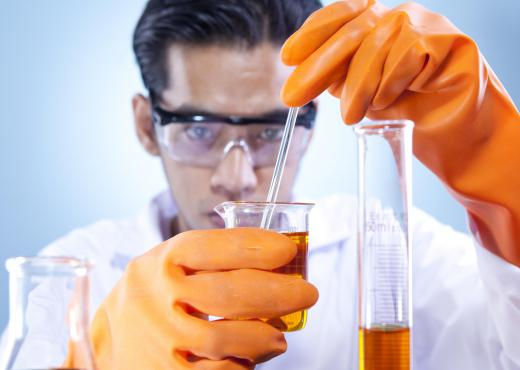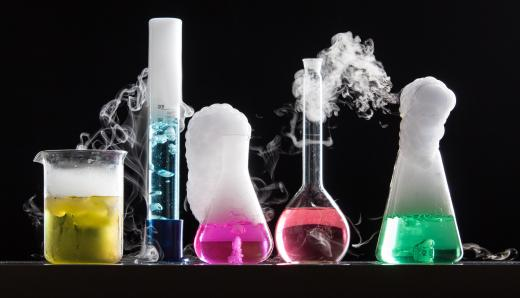Science
Fact Checked

# In Chemistry, what is a Limiting Reactant?

M.R. Anglin
M.R. Anglin

When a chemist wants to make a certain amount of a product, he needs to know how much of each chemical reactant to use. Similarly, if he has a certain amount of a reactant, it may be useful to find out how much product he will be able to make with it. In chemistry, one of the factors that a chemist will need to know in order to make or determine how much product he can make is the limiting reactant. The limiting reactant, also called a limiting reagent, limits the amount of product that can be made in a reaction—once this reactant is consumed, the reaction will stop. It is important, therefore, that the chemist know how to determine which reactant is the limiting reactant and to ensure he has enough of it to make the desired amount of product.

The limiting reactant is the reactant that will run out first as a reaction progresses. When the limiting reactant is used up, the reaction will cease. Any chemical or substance has the potential to be a limiting reactant. In order to determine which reactant is the limiting one, the chemist will need to determine how much of each substance he has. He will also need to know what proportion of each reactant the reaction needs in order to continue the desired amount of time.A chemist determines the limiting reactant by balancing the chemical equation.

A balanced equation can help a scientist know the proportion of each reactant. A balanced equation is one that reflects the law of conservation—nothing is created or destroyed during the reaction. In other words, there are as many atoms on one side of the equation as there are on the other. For instance, the balanced equation for making water is 2 H2 + O2 = 2 H2O. It is clear here that we need twice as many hydrogen atoms as oxygen atoms to make water.The limiting reactant limits the amount of product that can be made in a given reaction.

Before he can determine which reactant is the limiting reactant, a scientist needs to know how many moles of each substance he has. A mole is equal to approximately 6.02 x 1023 units of the substance and weighs the same as the molecular weight of that substance. For instance, since the molecular weight of hydrogen is approximately 2 grams, a mole of hydrogen molecules would also weigh approximately 2 grams and be roughly equal to 6.02 x 1023 molecules of hydrogen. Similarly, the molecular weight of oxygen— approximately 32 grams—is roughly equal to one mole of oxygen molecules. So, if the chemist has two grams of hydrogen and 32 grams of oxygen, he knows that he has about a mole of each substance.

Once the chemist has the proper balanced equation and knows how much of each reactant he has, he can then determine which reactant is the limiting reactant. For example, if the scientist determines he has one mole of hydrogen and one mole of oxygen, hydrogen would be the limiting reactant. Per the balanced equation for making water, you can see that it takes twice as many moles of hydrogen atoms than oxygen atoms to make water. In other words, each oxygen atom needs two hydrogen atoms in order to make water. The hydrogen would run out before the oxygen does, and once that happens, the reaction would stop.

## You might also LikeCertlerant

In a good business model it is important to have an idea of something like this. Waste is money thrown away. When making a product for sale, you need to make sure you don't waste the ingredients, you need to control your production on all levels.

If you have a mixture where you add more of certain chemical, and that chemical is not absorbed into a refined finished product. Your product is essentially watered down with wasted base chemical.

You want a well refined high quality product and eliminating waste is helpful with creating a high quality product.